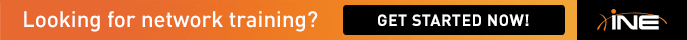# which rate-limit mode should be used in the exam ?

Hello

we have modes of CAR in Cisco routers , normal burst and extended burst

and i need to know which mode should be used in the exam if the task didn't guide me to spesific one ?

each has different formula to calculate CAR paramaters

## Comments

• If the task wasn't specific, then it really doesn't matter - just make something up.
I saw somewhere that a good formula is Bc = 1/8 of CIR. And often Be is configured the same as Bc.

So let's say you rate limit to 64K, then your Bc and Be will be 8K.

• this is normal burst formula BC=BE and BC=CIR/8

but extended burst formula is

BC = 1.5 X (CIR/8)

BE= 2 X BC

so when each should be used ?

• Normal burst formula is most common. I would use it.

Sign In or Register to comment.